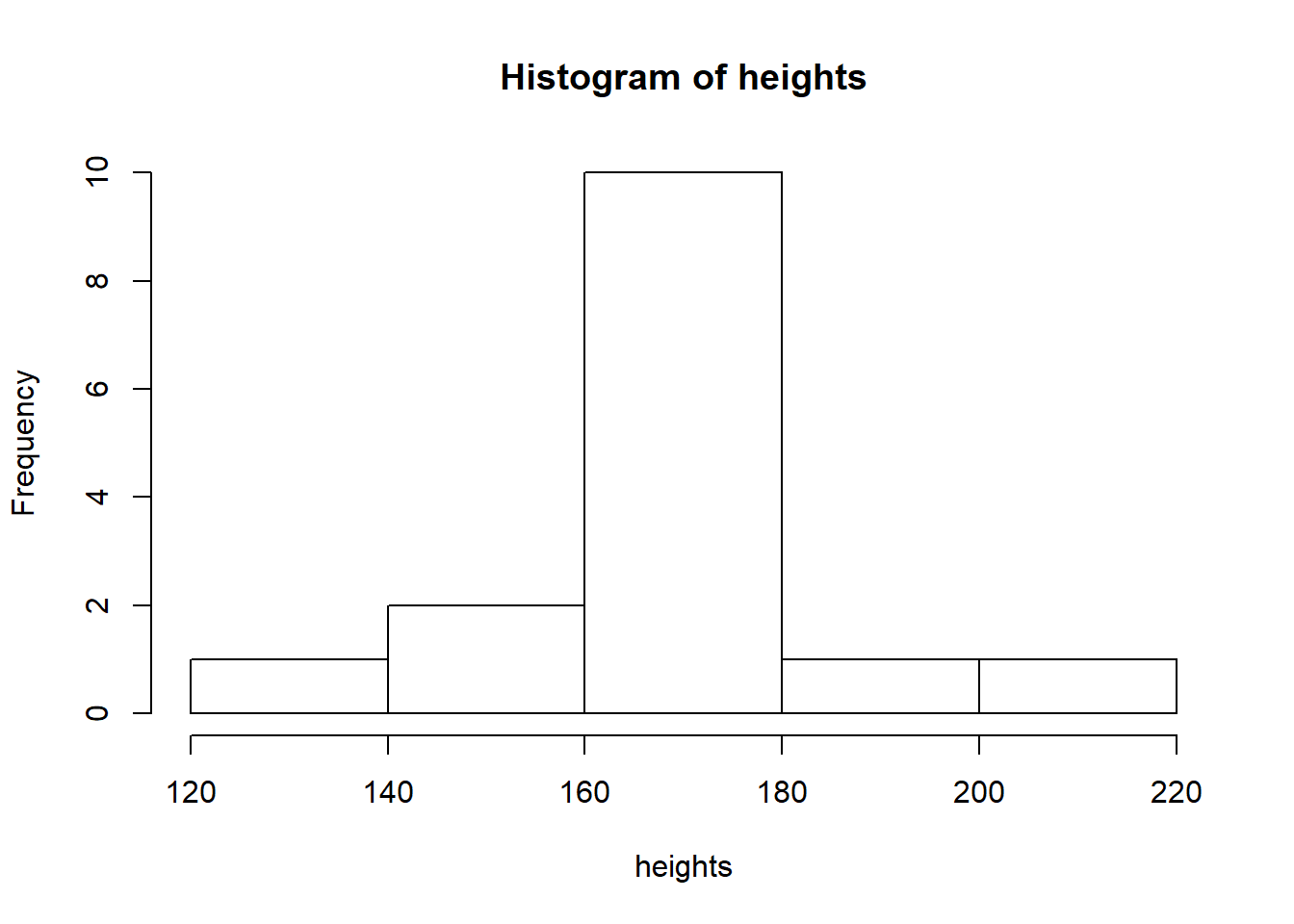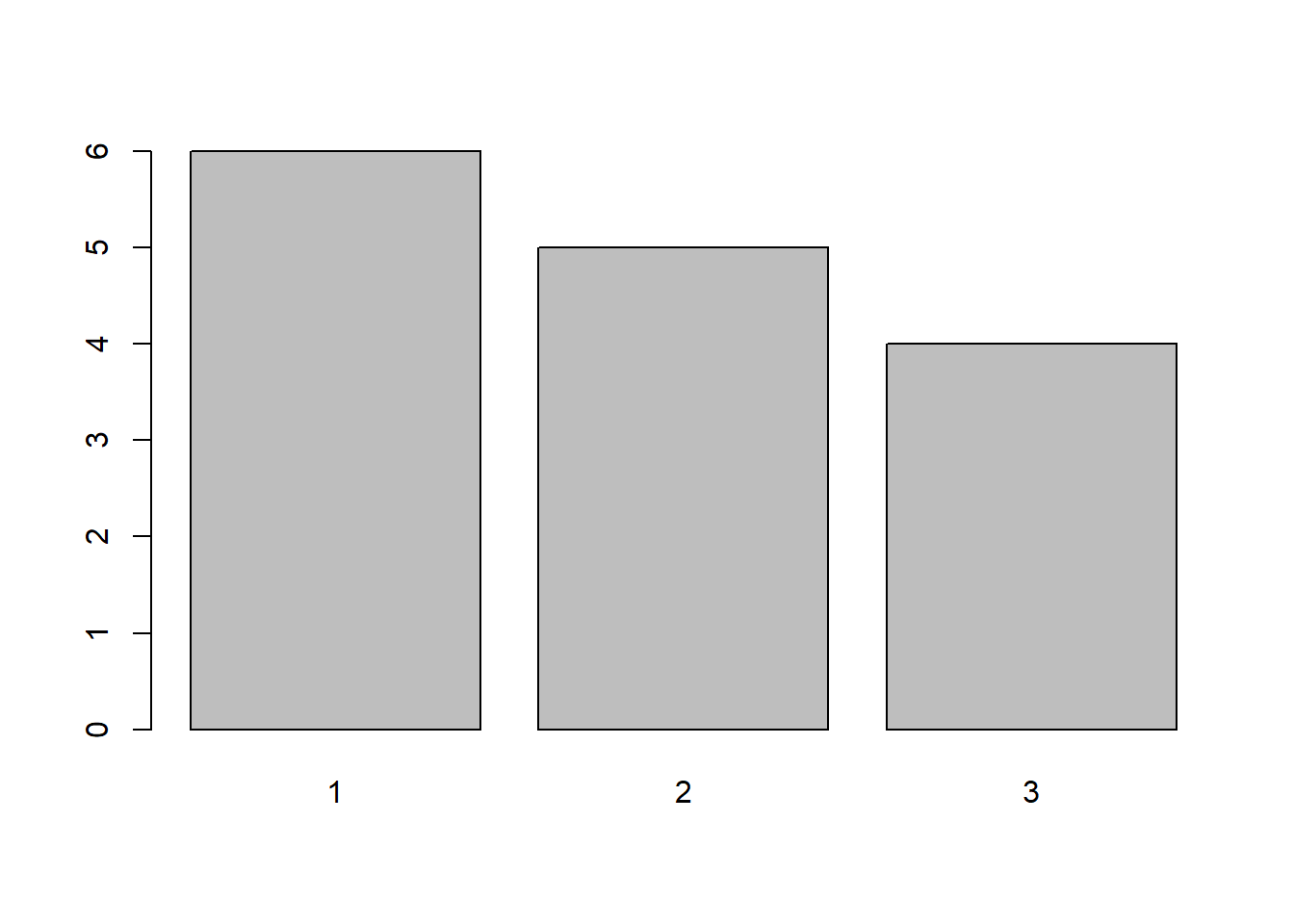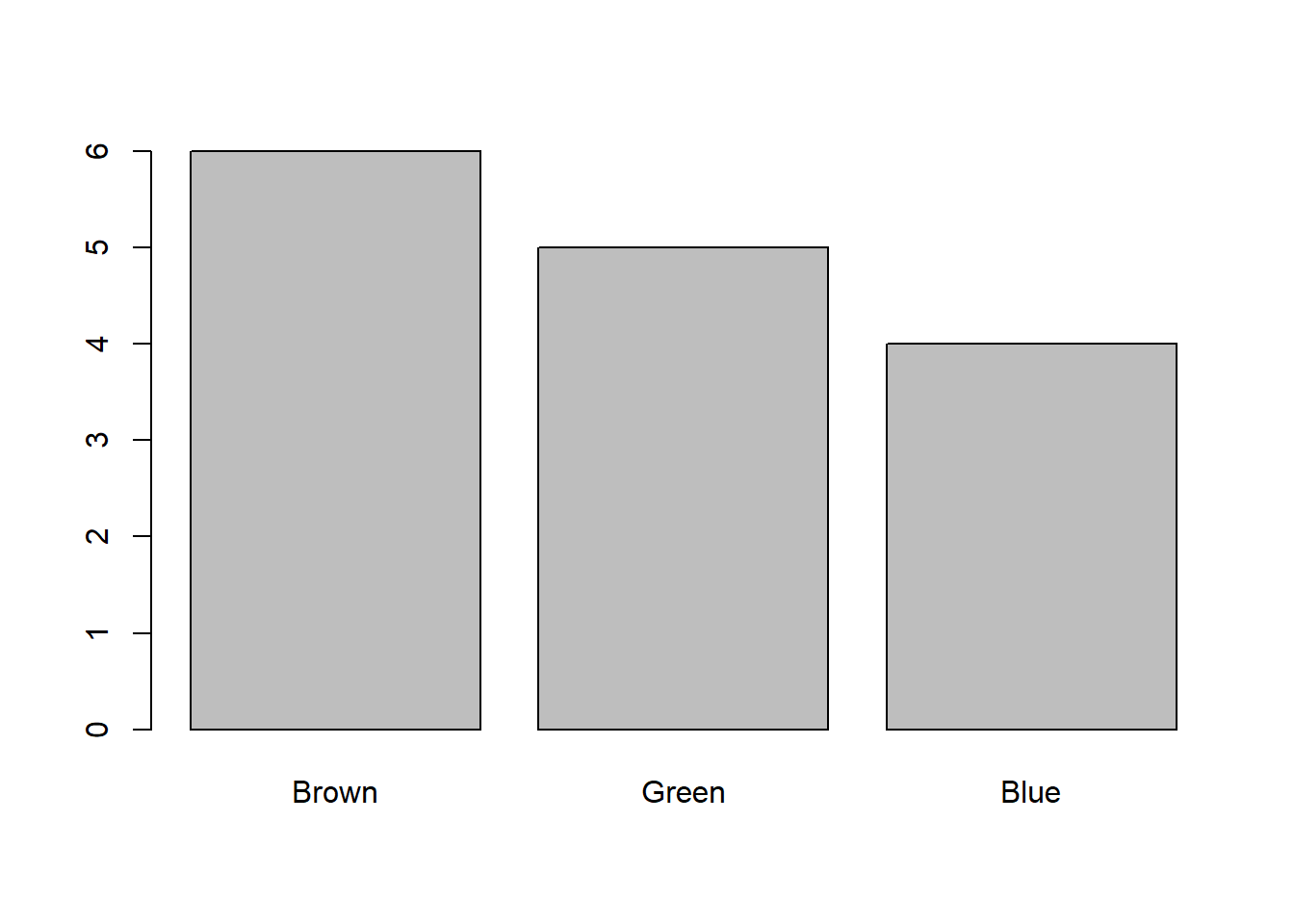### Class exercise

Note: the internet is full of good tutorials for learning R:

• In case you want to brush up on your R skills outside this course, good starting points can be found e.g. on RStudio’s online learning page.

• Problems related to the use of particular functions are often most efficiently solved by searching the R section of Stack overflow where someone else has with high probability encountered the same issue before.

• The internal documentation of R can also sometimes be helpful. To reach it, use either the help tab on the lower right corner of RStudio or directly ask for the help page of a particular function using e.g. ?rnorm or help(rnorm).

1. Visit the website https://data.oecd.org/ and pick three data sets that you find interesting. What kind of tools are used to summarize/plot the data? Are the summaries/plots clear and easy to read?
2. Search online for statistics about the income distribution in different countries. How are the data typically summarized/plotted? Are the general trends and patterns easy to spot from the used summaries/plots?

1. Generate a sample of 100 observations from the standard normal distribution and save it as the vector x
2. Calculate the sample mean and sample standard deviation of x using the functions mean and sd.
3. Find (or code!) a function that will compute the sample variance of a vector of values.
4. Generate three samples from a normal distribution with expected value 1 and standard deviation 3, one with 10 observations, one with 100 and one with 1000.
5. Compute the sample means and sample standard deviations of the three samples in part d. How do the statistics behave when the sample size is increased? What causes this?
# a.
x <- rnorm(100) # Or, more explicitly, x <- rnorm(100, 0, 1)

# b.
mean(x); sd(x)
##  -0.2536933
##  1.121438
# c.
var(x)
##  1.257622
# d.
x_10 <- rnorm(10, 1, 3) # Note that rnorm takes standard deviation, not variance, as its argument
x_100 <- rnorm(100, 1, 3)
x_1000 <- rnorm(1000, 1, 3)

# e.
mean(x_10); sd(x_10)
##  1.45416
##  3.299392
mean(x_100); sd(x_100)
##  1.482397
##  2.79772
mean(x_1000); sd(x_1000)
##  0.8295466
##  2.998261
# With increasing sample size, the statistics converge to their population counterparts by the law of large numbers.

1. Collect together a random sample, 10-15 observations, of the heights of the students in the class and save the data as a vector.
2. Explore how the heights of the students are distributed by finding out how the function hist works and drawing a histogram of the heights.
3. How do you think the histogram would change if we had sampled the whole classroom? How about if we had sampled 2000 random students from the Aalto university?
4. Find out how to change the number of bins in the histogram and experiment with it to see how the plot changes.
# a.
heights <- rnorm(15, 170, 15) # Fill in here.

# b.
hist(heights)

# c.
# The histogram would most likely approach the true distribution of all students in Aalto university. Human height is often well approximated by the normal distribution, and assuming that this holds for the student population of Aalto University, at least in the 2000 student sample the resulting histogram would most likely look like something resembling a normal distribution. However, even if this holds, the histograms of the classroom samples can still look quite non-normal as the sample sizes are small (and the classroom sample might not be representative of the whole Aalto student population).

# d.
# The number of bins (breakpoints) is controlled by the argument "breaks"
hist(heights, breaks = 3)# For small sample sizes, the histograms can change quite a lot when the number of bins is varied. 

1. Collect together a random sample, 10-15 observations, of the eye colors of the students in the class and save the data as a vector. Code the different colors either using different numbers (for example, blue is 1 etc.) or, if you know how, using the factor class in R.
2. Find out which function draws a bar chart and use it to plot the data. The function table might also prove useful.
3. How do you think the bar chart would change if we had sampled the whole classroom? How about if we had sampled 2000 random students from the Aalto university?
# a.
eyecolors <- sample(1:3, 15, replace = TRUE) # Fill in here.
eyecolors_2 <- factor(eyecolors, labels = c("Brown", "Green", "Blue"))

# b.
barplot(table(eyecolors))barplot(table(eyecolors_2))# c.
# See answer c. in the previous problem. Here the true distribution is a discrete distribution of all possible eye colors encountered amongst Aalto students. Also, sampling a larger population could reveal some rare eye colors that are not found in the sample, thus adding more bars to the chart.

1. (Optional) Install both R and RStudio on your personal computer and use them to experiment with the lecture and exercise codes throughout the course. If you have troubles in the installation, you can bring your laptop with you to the next exercise session and ask the course assistant to help you.

1. (Optional) The RStudio website is a home to numerous useful cheat sheets which list the key commands of various packages and tasks (plotting, data import etc.) Check them out, paying attention especially to the “RStudio IDE Cheat Sheet”.

1. (Optional) If you prefer learning R hands-on, check out the R-package swirl, a real-time tutorial of R inside R.
install.packages("swirl")
library(swirl)
swirl()

1. (Optional) This exercise sheet was created using R Markdown. Try it out yourself by choosing “File -> New File -> R Markdown…” in RStudio. Try “knitting” the document into a .html of .pdf file by pressing the Knit button in the toolbar. Use the R Markdown Cheat Sheet to experiment with different formatting in your R Markdown document.